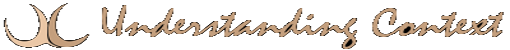# 17 Jan Perceptrons and Weighted Schemes

###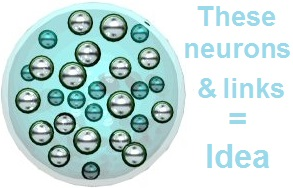Perceptrons

In the late 1600’s, John Locke expounded an associationist theory in which neurons or “bundles” of neurons came to represent certain ideas and associations between ideas. Rosenblatt‘s work seems a logical extension of associationist theory. Perceptrons can perform linear discrimination, thus enabling them to model the cognitive function of recognition (or, in computational terms, pattern classification). In other words, given an input pattern X, a perceptron can determine if it is a known pattern. The discriminators in the model are weights between the input channel and the output element. If, on a given input, the formula for calculating the product of the input vector and the weight vector yields a +1, then the pattern is recognized. Otherwise, the product will be -1. There is no explicit representation of the “learned” data in the system.

Understanding Context Cross-Reference
Click on these Links to other posts and glossary/bibliography references
 Prior Post Next Post Roots of Neural Nets Multi-Layered Perceptron Definitions References associationism  spreading activation Locke 1689 Rosenblatt 1958 Minsky 1975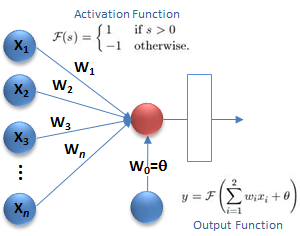Many ANS use the results of Rosenblatt’s work. The “tendency of similar stimuli to activate the same set of cells,” Rosenblatt’s fifth assumption, has been translated into a model with many interconnected processors, or processing elements (PEs), that form a network in which input stimuli (voltages) flow in one direction through the network, gaining and losing intensity as the result of the “strength” or weights of links between PEs. The formula for a linear discriminant over class C is:

A perceptron model of linear discrimination is shown in the figure at right.

Given input pattern X and vector weight W with the activation function, produce output y using a formula for averaging the weights:

### Weights

While we are talking about weights, let us draw a parallel between physiological structures and cognitive processes. The parallel is between the thresholds of E/I activation that travel through the brain and the thresholds of belief that travel through the mind. This may be an artificial metaphor, but, on the other hand, it may lead us down new paths worth exploring.

The mathematical process of using threshold logic to make a binary decision is exactly analogous to the physiological process of spreading activation. In the case of one neuron, we have a do-or-die algorithm. If we look at more than one neuron or look for “heated up” regions (or bundles) of neurons, we could describe the algorithm in fuzzier terms of deciding whether or not to make decision A (see Mathematical Process at left). The mathematical and cognitive models can also represent a variety of decisions based on pre-established threshold parameters. In our mechanical brain, we can place algorithms of the sort described in the colored boxes at multiple levels.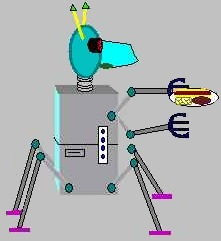When MIPUS has to decide whether or not to begin clearing dishes from the table, he must go through a process of weighing many different factors he has observed. He should not begin until the aggregate weight of the factors exceeds the “clear the table” threshold.

### Logical Processor algorithm:

1. if weight(X) > threshold(T) then
• execute decision(A)
2. else
• do not execute decision(A)
3. end if

### Physiological Process:

1. if Accumulated input >-40mV then
• propagate action potential
2.else
3. end if

### Cognitive Process:

1. ifIbelieveinput to be true then
• act on my belief
2. else
• disregard input
3. end if

### Weights Illustrated

A simplified model of weights is shown in the illustration above. In this model, each neurode has an output weight that applies to all outputs. More complex models have different weights for each output. In neurons, the level of chemicals at a synapse combined with the level of excitation or inhibition and other factors determines whether or not E/I will cause the synapse to fire and propagate the impulse to the next neuron. This weighting system models that phenomenon.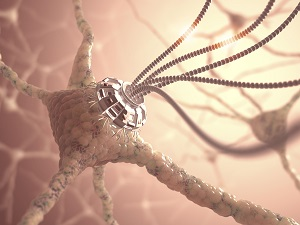Remember that perceptrons were designed for a mechanical form of recognition called pattern classification. ANS are designed so that they can both perform pattern classification and learn new patterns. This learning process is called adaptation, and it involves automatically changing the weights of links between neurodes (like perceptrons). The theoretical underpinnings of adaptive pattern classification can be found in probability theory, Bayesian decision theory, and feature vectors or feature space. Using these mathematical models, algorithms can be developed to automatically adjust weights in the system by comparing the output with the desired output and propagating change factors backward through the network of neurodes and links.

Here are a couple of good videos illustrating the process of spreading activation and Nick Unick’s video and Leslie Samuel’s video.

Click below to look in each Understanding Context section
 Intro Context 1 Brains 2 Neurons 3 Neural Networks 4 Perception and Cognition 5 Fuzzy Logic 6 Language and Dialog 7 Cybernetic Models 8 Apps and Processes 9 The End of Code Glossary Bibliography